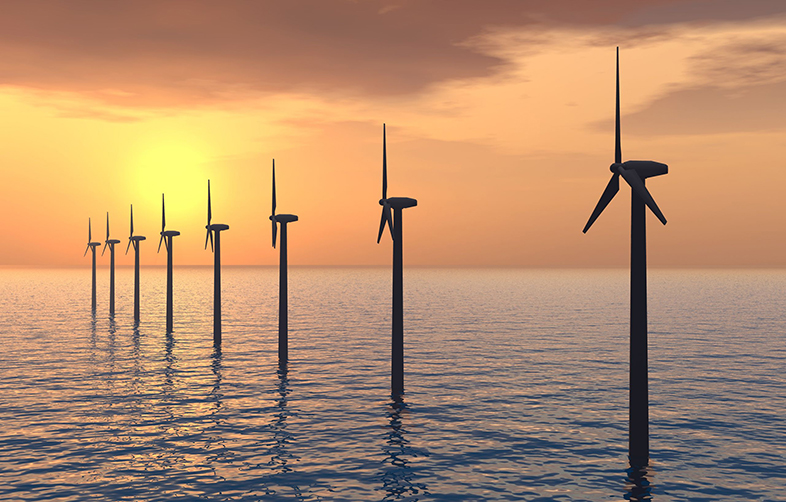Science, Maths & Technology

### Become an OU studentCan renewable energy sources power the world?

Start this free course now. Just create an account and sign in. Enrol and complete the course for a free statement of participation or digital badge if available.

# 2.2 Efficiency and capacity factor

When energy is converted from one to another, what comes out is never as much as what goes in. The ratio (usually expressed as a percentage) is called the efficiency of the process:

percentage efficiency = (energy output/energy input) x100

Some types of energy conversion can be really efficient, for example up to 90% in a water turbine; others very low - around 10-20% in a typical internal combustion engine.

If you’re trying to assess an energy generator’s productivity in practice, one useful measure is its capacity factor (CF):

capacity factor = actual energy output over time / maximum possible output

The period of time can be a year, a month, a week or an hour. The units for the output quantities can be kWh, MWh, GWh, etc., and the capacity factor can be expressed as either a fraction or a percentage. The time period to which the capacity factor relates (a year, a month, a week etc.) should be stated.

Have a look at a couple of examples:

## Activity 1

What would be the annual capacity factor of a 1 MW plant running constantly at a full rated capacity for one year?